Next: 6.4 Numerical Calculations: Solid Up: 6 Quantum Diffusion II: Previous: 6.2 Muonium Diffusion in

# 6.3 Experimental Results in Solid Nitrogen

In solid nitrogen muonium diffusion was studied using both longitudinal and transverse field muon spin rotation/relaxation techniques. From the relaxation rates of the muon polarization one can extract the muonium hop rate as a function of temperature. This is compared to the behavior predicted by theory, finding agreement at low temperatures only.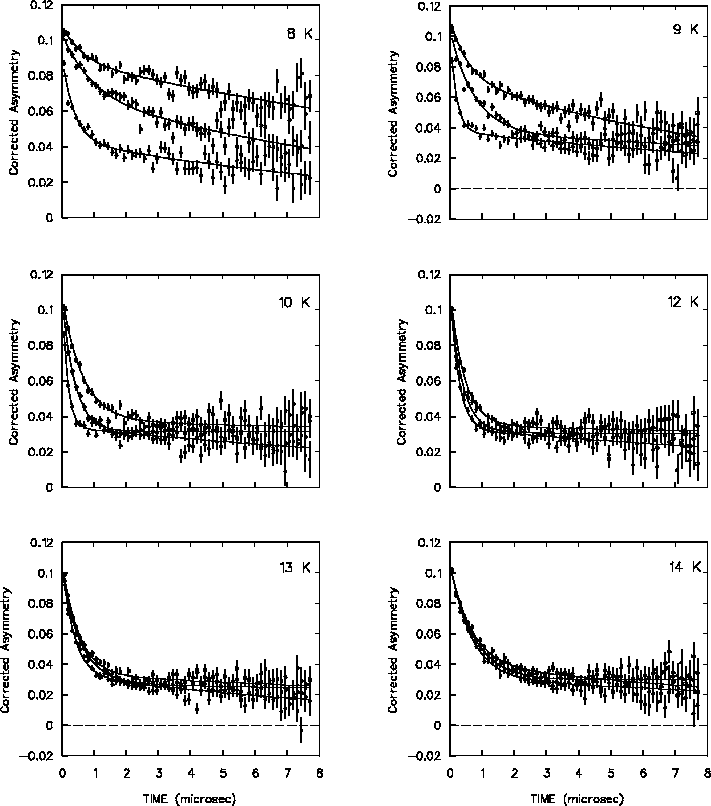With a weak longitudinal magnetic field of a few Gauss applied to the sample, the muon and electron spins in muonium are strongly coupled by the hyperfine interaction. As the muonium atom diffuses among atoms of the host lattice, the nuclear hyperfine (nhf) interaction between the spins of the muonium electron and the randomly-oriented lattice nuclear moments undergoes fluctuations. The resulting time-dependent effective magnetic field felt by the muonium atom excites transitions among states of the muonium atom, and the original polarization of the muon spin is lost. Figure 6.18 shows the temperature and magnetic field dependence of the muon asymmetry measured in s-N2in longitudinal field. To a good approximation, in weak magnetic fields the muon spin polarization relaxes with an exponential time dependence

 Pz(t)=Pz(0) e-t/T1 (11)

where the relaxation rate 1/T1 depends on the hop rate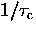according to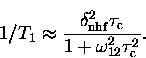(12)
Equation (6.13) also predicts the presence of a 1/T1 maximum where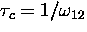.Such maxima are evident in the relaxation rates, which peak at different temperatures depending on the field, allowing one to make an unambiguous determination of the diffusion rate independent of the absolute scale of the relaxation rate.

Figure  6.19 shows examples of muonium spin precession signals measured in a weak transverse field (wTF) of 5 G. In weak magnetic fields the two observable muonium frequencies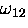and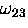are very nearly equal (see Appendix A for definitions of these and the other muonium frequencies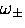and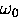)the splitting between these two lines is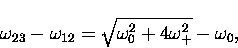(13)
and in fields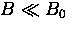this is approximately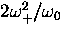.In a transverse magnetic field of 8 G or less, and when the muonium relaxation rate is greater than about 0.25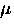s-1, beating'' of the two triplet muonium frequencies is not apparent within the 10s time range of the histogram; the muonium spin precesses with one signal at the mean frequency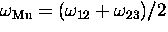= 1.3961 MHz/G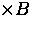.(However, in slightly larger magnetic fields, or if the spin relaxation rate is extremely low, the beat envelope will masquerade as a Gaussian relaxation function which, if one mistakenly fitted the asymmetry with a single signal, would result in a misleading contribution to the real relaxation rate.) The asymmetry measured in s-N2 was fitted to a sum of signals for fast and slowly relaxing diamagnetic fractions and the muonium fraction: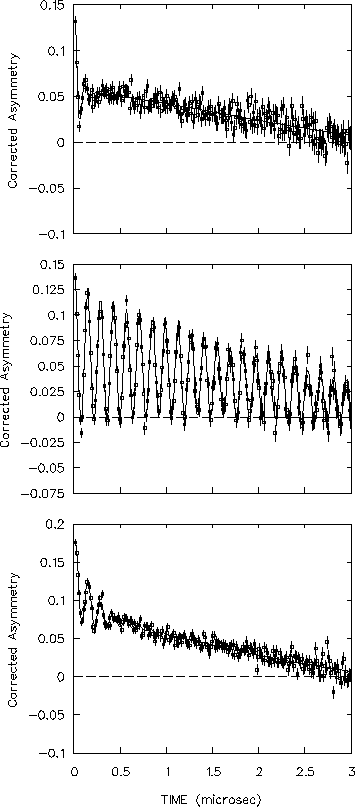In transverse field the polarization relaxation function depends on the particle hop rate due to the motional narrowing of the precession frequency linewidth. Assuming that the local (effective nhf) field correlation function is described by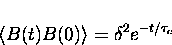(14)
(where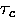is the correlation time and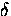is the width of the field distribution) the relaxation function is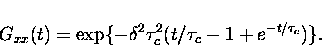(15)
In the case of sufficiently slow hopping when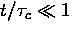,the TF relaxation function approaches the Gaussian form of the static limit

independent of, with relaxation rate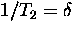.In the case of fast hopping the effective nhf field is averaged over many sites resulting in the motional narrowing of the linewidth; the relaxation function tends to an exponential form:

with the smaller relaxation rate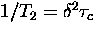. In the limit of fast hopping the TF and LF experiments should give the same relaxation rates. Figure 6.20 shows examples of the relaxation function for several correlation times corresponding to mean hop rates from 0 to 50s-1.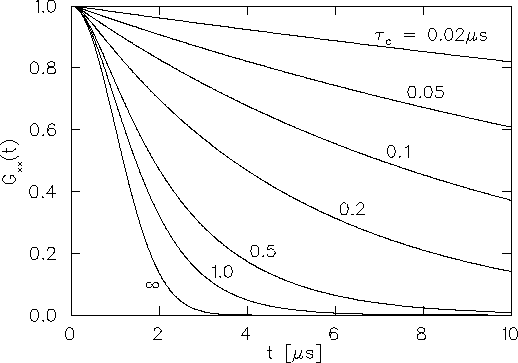Figure 6.21 shows the muonium spin relaxation rates measured in s-N2by TF and LF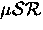experiments. The qualitative temperature dependence of the relaxation rates and the hop rate is entirely consistent with the following interpretation. At low temperatures (T < 5K) muonium is nearly static; the TF experiment measured a T-independent relaxation rate as expected in the slow-hopping limit. Between about 9 K and 20 K the TF relaxation rate drops, as expected if the muonium diffuses faster as temperature rises. The LF relaxation rates in 4, 8 and 12 G reach their maxima, where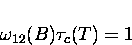is satisfied, at progressively higher temperatures. Between about 11 K and 15 K the relaxation rate in LF becomes field-independent and approaches the TF relaxation rate, also indicating that the hop rate increases with temperature in this part of the data.

Making use of Eqs. (6.13),(6.18) and (6.19) we can extract the muonium hop rate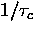as a function of temperature, as shown in Fig. 6.22. The LF experiments measured a diffusion rate increasing as T6.7(1) up to 15 K. The TF data extend this to higher temperatures where the correlation timeis so short that the LF relaxation rate becomes nearly independent of magnetic field and approaches the TF relaxation rate. Between about 20 and 30 K the hop rate reaches its maximum; the TF relaxation rate become T-independent at about 0.4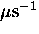.If the hop rate here is limited only by the coherent tunnelling bandwidth, we can estimate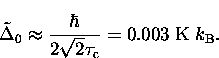It is also possible that the relaxation rate due to the motionally averaged nuclear hyperfine interaction with N2moments is even smaller, but is overwhelmed by spin relaxation due to other causes, such as the muonium atoms diffusing to chemically active impurities or impurites with electronic moments such as O2 that immediately depolarize any muonium atom that strays near. Above about 50 K the TF relaxation rate increases as the hop rate drops with increasing temperature, a key characteristic of incoherent (2-phonon) quantum diffusion, although theory predicts a much weaker temperature dependence at such a large fraction of the Debye temperature.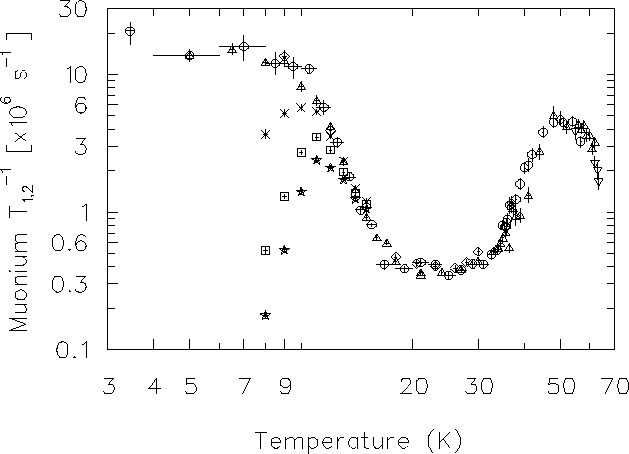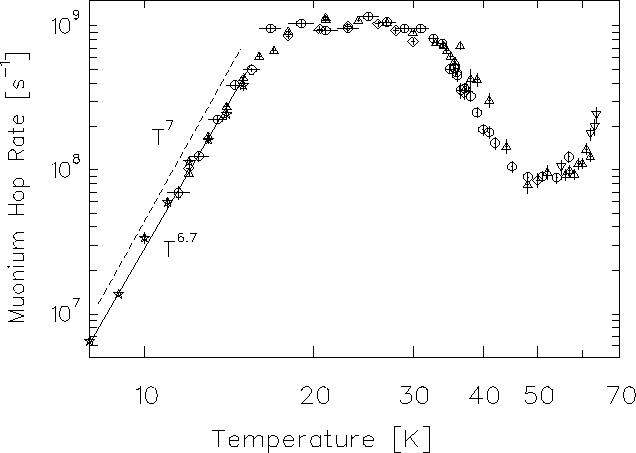Next: 6.4 Numerical Calculations: Solid Up: 6 Quantum Diffusion II: Previous: 6.2 Muonium Diffusion in